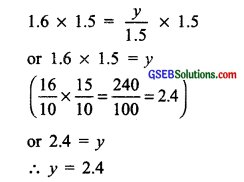# GSEB Solutions Class 8 Maths Chapter 2 Linear Equations in One Variable Ex 2.1

Gujarat Board GSEB Textbook Solutions Class 8 Maths Chapter 2 Linear Equations in One Variable Ex 2.1 Textbook Questions and Answers.

## Gujarat Board Textbook Solutions Class 8 Maths Chapter 2 Linear Equations in One Variable Ex 2.1

Question 1.
Solve the following equations.
(i) x- 2 = 7
(ii) y + 3 = 10
(iii) 6 = z + 2
(iv) $$\frac { 3 }{ 7 }$$ + = $$\frac { 17 }{ 7 }$$
(v) 6x = 12
(vi) $$\frac { t }{ 5 }$$ = 10
(vii) $$\frac { 2x }{ 3 }$$ = 18
(viii) 1.6 = $$\frac { y }{ 1.5 }$$
(ix) 7x – 9 = 16
(x) 14y – 8 = 13
(xi) 17 + 6p = 9
(xii) $$\frac { x }{ 3 }$$ + 1 = $$\frac { 7 }{ 15 }$$
Solutions:
(i) x- 2 = 7
Transposing (-2) to RHS, we have
x = 7 + 2
∴ x = 9(ii) y + 3 = 10
Transposing 3 to RHS, we have
y = 10 – 3
∴ y = 7

(iii) 6 = z + 2
Transposing 2 to LHS, we have
6 – 2 = z
or 4 = z
∴ z = 4

(iv) $$\frac { 3 }{ 7 }$$ + = $$\frac { 17 }{ 7 }$$
Transposing $$\frac { 3 }{ 7 }$$ to RHS, we have
x = $$\frac { 17 }{ 7 }$$ – $$\frac { 3 }{ 7 }$$ = $$\frac { 17 – 3 }{ 7 }$$ = $$\frac { 14 }{ 7 }$$ = 2
∴ x = 2

(v) 6x = 12
Dividing both sides by 6, we have:
$$\frac { 6x }{ 6 }$$ = $$\frac { 12 }{ 6 }$$ or x = 2
∴ x = 2(vi) $$\frac { t }{ 5 }$$ = 10
Multiplying both sides by 5, we have
$$\frac { t }{ 5 }$$ ×5 = 10 ×5 or t = 50
∴ t = 50

(vii) $$\frac { 2x }{ 3 }$$ = 18
Multiplying both sides by 3, we have
($$\frac { t }{ 5 }$$) × 3 = 18 × 3 or 2x = 54
Dividing both sides by 2, we have
$$\frac { 2x }{ 2 }$$ = $$\frac { 54 }{ 2 }$$
or x = $$\frac { 54 }{ 2 }$$ = 27
∴ x = 27(viii) 1.6 = $$\frac { y }{ 1.5 }$$
Multiplying both sides by 1.5, we have(ix) 7x – 9 = 16
Transposing (-9) to RHS, we have
7x = 16 + 9 = 25
Dividing both sides by 7, we have $$\frac { 7x }{ 7 }$$ = $$\frac { 25 }{ 7 }$$
∴ x = $$\frac { 25 }{ 7 }$$

(x) 14y – 8 = 13
Transposing -8 to RHS, we have
14y = 13 + 8 = 21
Dividing both sides by 14, we have
$$\frac { 14y }{ 14 }$$ or y = $$\frac { 21 }{ 14 }$$ = $$\frac { 3 }{ 4 }$$
∴ y = $$\frac { 3 }{ 2 }$$(xi) 17 + 6p = 9
Transposing 17 to RHS, we have
6p = 9 – 17 = -8
Dividing both sides by 6, we have
$$\frac { 6p }{ 6 }$$ = $$\frac { -8 }{ 6 }$$ or p = $$\frac { -8 }{ 6 }$$ = –$$\frac { 4 }{ 3 }$$
∴ p = $$\frac { 4 }{ 3 }$$(xii) $$\frac { x }{ 3 }$$ + 1 = $$\frac { 7 }{ 15 }$$
Transposing 1 to RHS, we have
$$\frac { x }{ 3 }$$ = $$\frac { 7 }{ 15 }$$ – 1
or $$\frac { x }{ 3 }$$ = $$\frac { 7 – 15 }{ 15 }$$ = $$\frac { -8 }{ 15 }$$

Multiplying both sides by 3, we have
$$\frac { x }{ 3 }$$ ×3 = $$\frac { -8 }{ 15 }$$ ×3 = – $$\frac { -8 }{ 5 }$$
∴ x = $$\frac { -8 }{ 15 }$$